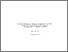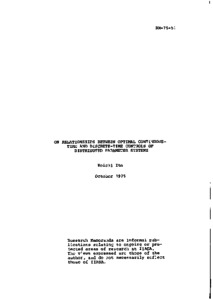# On Relationships Between Optimal Continuous-Time and Discrete-Time Controls of Distributed Parameter Systems

Ito, K. (1975). On Relationships Between Optimal Continuous-Time and Discrete-Time Controls of Distributed Parameter Systems. IIASA Research Memorandum. IIASA, Laxenburg, Austria: RM-75-051Preview
Text
RM-75-051.pdf

## Abstract

In this paper, for a distributed parameter system described by a partial differential equation of parabolic type, two optimal control problems are investigated. From the engineering standpoint on the construction of control devices, we assumed that both distributed and boundary controls are respectively concentrated spatially onto some spatial domains or onto some parts of the boundary. First, for a performance index in quadratic form, an optimal control problem with continuous-time control is considered. Applying the technique of dynamic programming, a non-linear integro-partial differential equation analogous to the Riccati equation has been obtained. Second, using Green's function representation, the same optimal control problem with discrete-time control is discussed, and the recurrence relationships to determine the optimal control policy have been derived. Lastly, relationships between the above-obtained optimal continuous-time and discrete-time controls have been discussed.

Item Type: Monograph (IIASA Research Memorandum) Management and Technology Area (MMT) IIASA Import 15 Jan 2016 01:42 27 Aug 2021 17:08 http://pure.iiasa.ac.at/465View Item